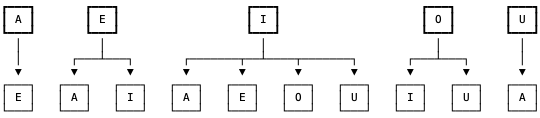Leetcode 1220：统计元音字母序列的数目（超详细的解法！！！）
in leetcode with 0 comment

• 字符串中的每个字符都应当是小写元音字母（'a', 'e', 'i', 'o', 'u'
• 每个元音 'a' 后面都只能跟着 'e'
• 每个元音 'e' 后面只能跟着 'a' 或者是 'i'
• 每个元音 'i' 后面 不能 再跟着另一个 'i'
• 每个元音 'o' 后面只能跟着 'i' 或者是 'u'
• 每个元音 'u' 后面只能跟着 'a'

输入：n = 1

输入：n = 2

输入：n = 5

• 1 <= n <= 2 * 10^4class Solution:
def countVowelPermutation(self, n: int) -> int:
a, e, i, o, u = 1, 1, 1, 1, 1
for _ in range(n - 1):
a, e, i, o, u = e + i + u, a + i, e + o, i, i + o
return (a + e + i + o + u) % (10**9 + 7)

reference:

https://leetcode.com/problems/count-vowels-permutation/discuss/398286/Simple-Python-(With-Diagram)

「如果我的文章对你有很大帮助，那么不妨～！」Responses